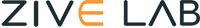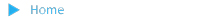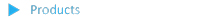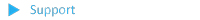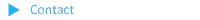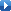ZMAN homepage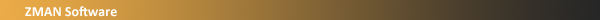Version 2.5

Unique Function in ZMAN

Automatic Model Searching
Automatic Initial Guessing

Parameter Plot
Impedance Parameter SimulationFeature

 Model simulation and fitting 2D- and 3D-Bode- and Nyquist plots Automatic equivalent circuit model search function Project concept to handle multiple EIS data analysis Parameter plot from fitted elements value Compatible with data format from Gamry, PGamry, Palmsens.Zahner, autolab,Biologic,Ametek HP, inphase,ivium,solatron,voltalab, ascii etc (needs License code Various weighting algorithm Model library and user model KK plot Batch fitting for project data Impedance parameter simulation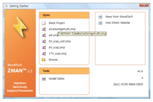Note: License code is not compatible with older versionWhat's NEW in ver. 2.2 f2

 R,C R,L R,Q preview & graphic ZHIT function Mott-schottky analysis Donor density vs. Vfb graph C vs. voltage graphWhat's NEW in ver. 2.2

 Interpolate bad data Black-Nichols plot 3D graph setting option Improved model editor Application model library for automatic searching Parameter simulation of model Genetic algorithm option for initial guessing Automatic initial guessing Trace movie function of fittingWhat's NEW in ver. 2.1

 Free for WonATech's & Zivelab's data format (*.wdf, *.wis) analysis (No license code required) Circle fitting Data editing available (Insert, Delete, Edit) Add/subtract element parameters Add/subract model parameters Impedance, Z in polar, admittance, Y in polar, modulus, M in polar, dielectric constant,E in polar, data display Empty cell capacitance calculation Find file function Data replacement by formula function Cursor data display Model finding result automatic sorting by Chi square value

A) R,C in R l l C (parallel connection)

a-1) Data display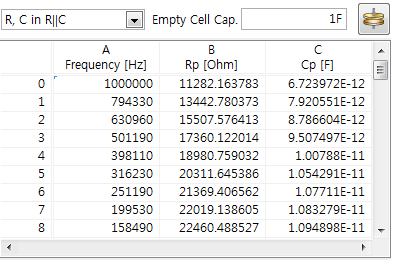a-2) Graphic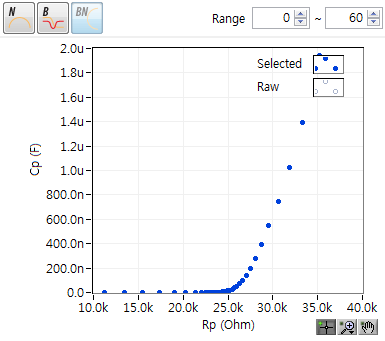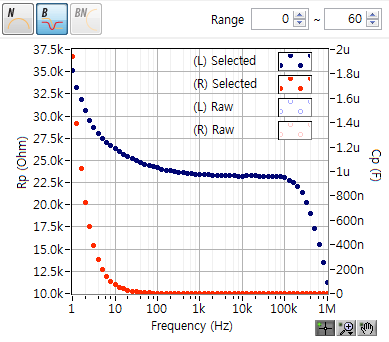B) R,C in R - C (serial connection)

b-1) Data display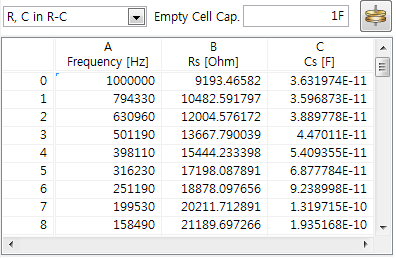b-2) Graphic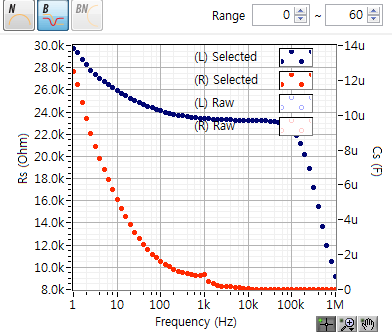C) R,L in R - L

c-1) Data display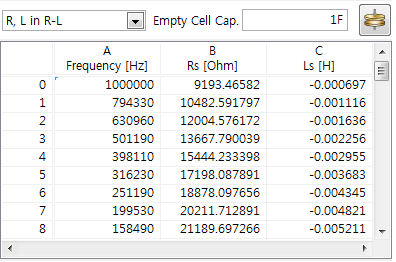c-2) Graphic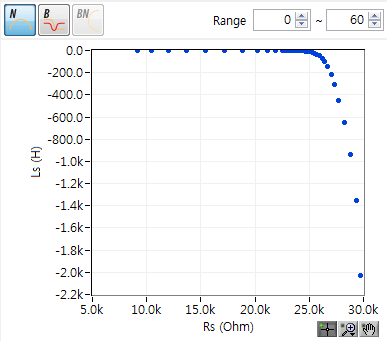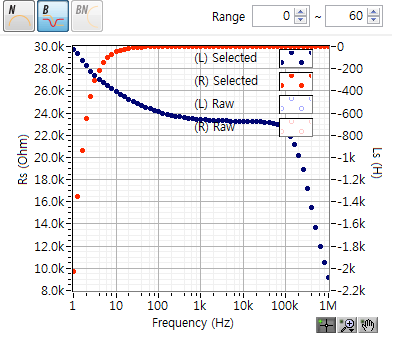D) R,Q in R - Q

d-1) Data display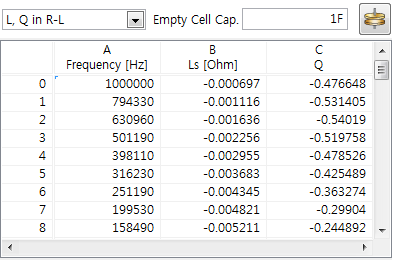d-2) Graphic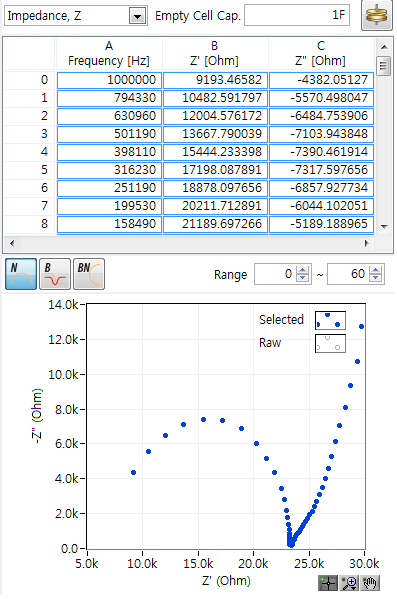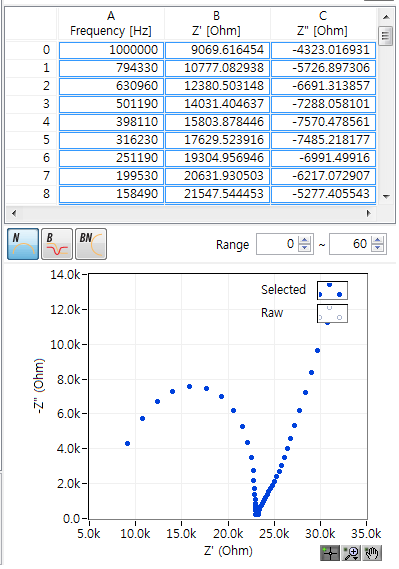Before (left) & after ZHIT (right)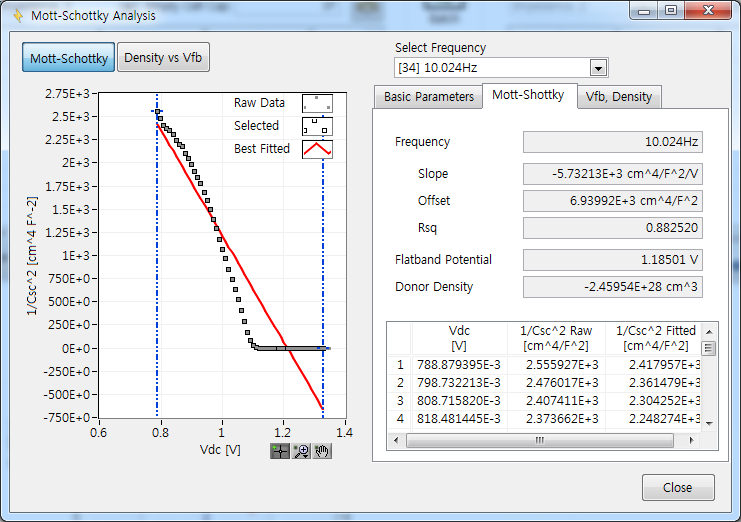Mott-Schottky analysis window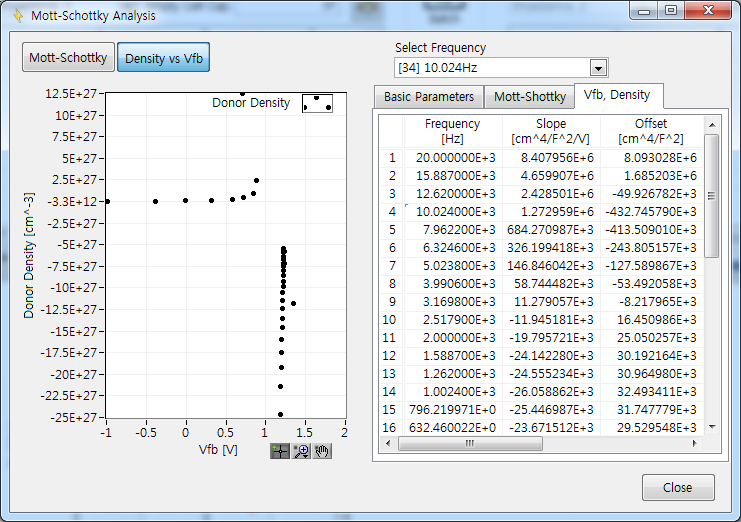Donor density vs. graph and analysisC/R-V graph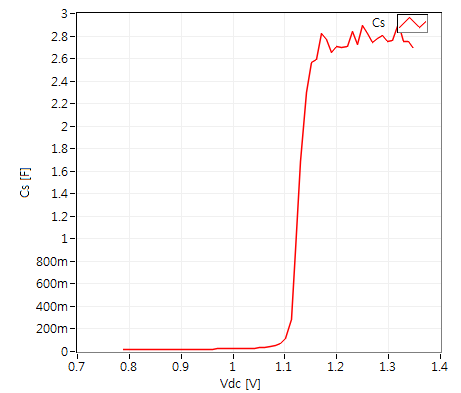Cp vs. voltage graph (parallel capacitance) Cs vs. voltage graph (serial capacitance)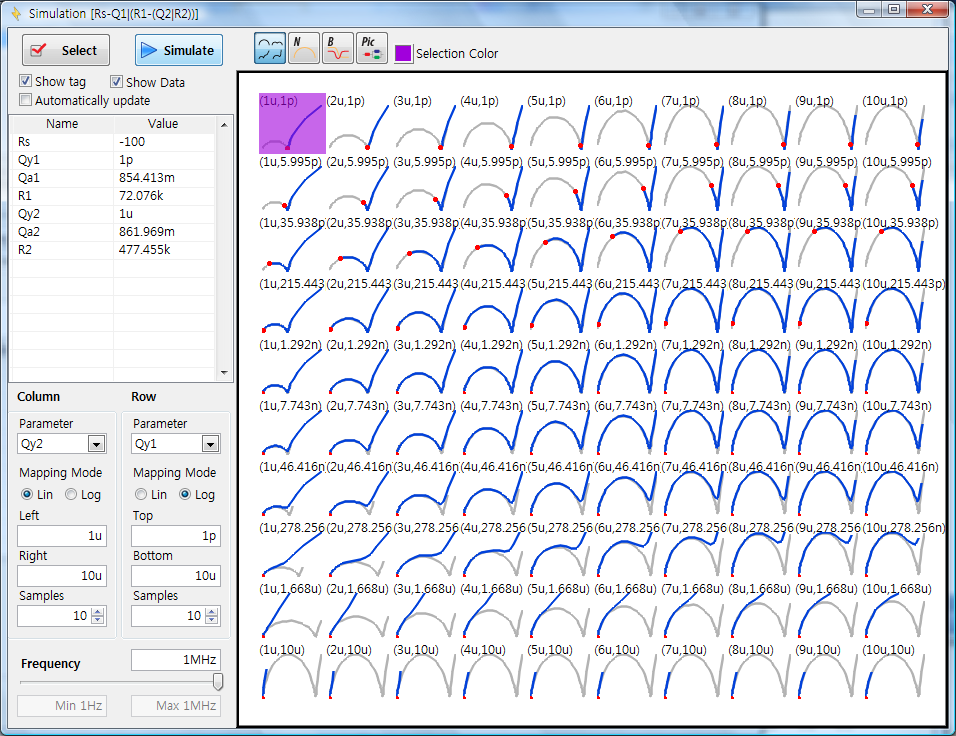Parameter simulation (Ver2.2)Circular fitting (Ver2.2)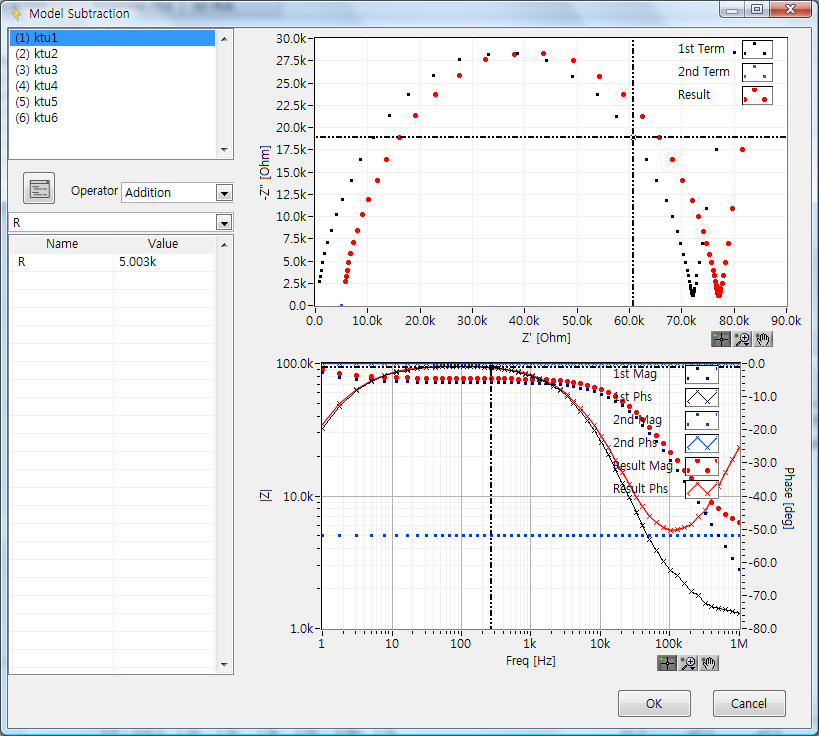Element add/subtraction (Ver2.2)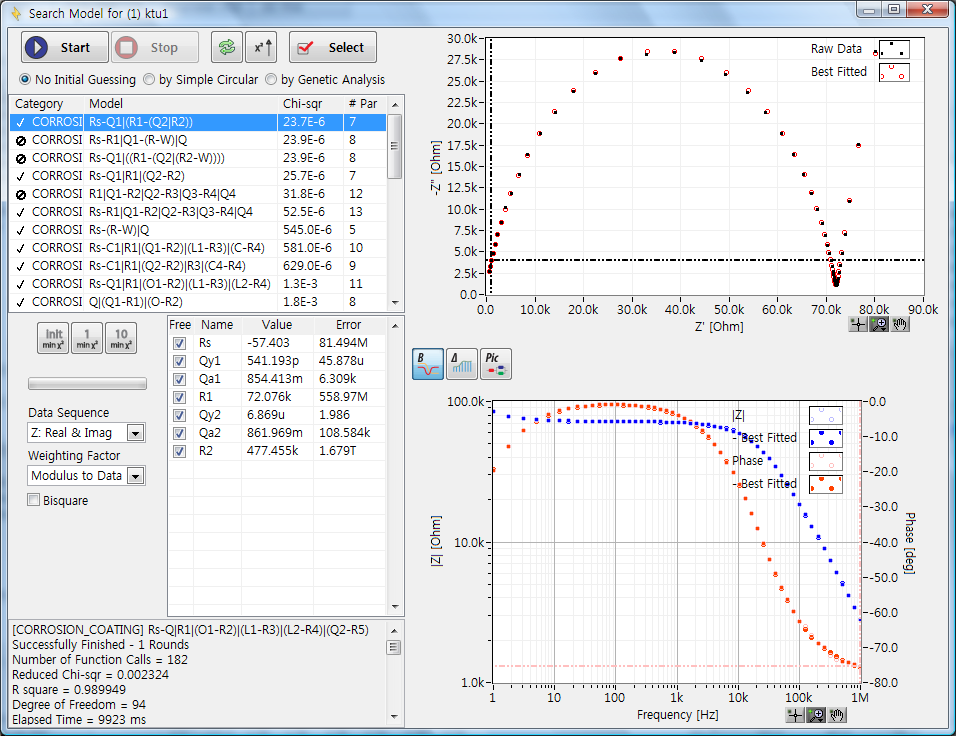Model finding result automatic sorting by Chi square value (Ver2.2)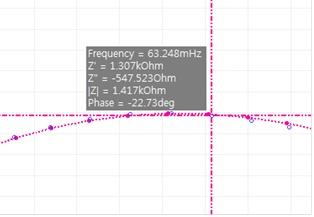Cursor data display (Ver2.2)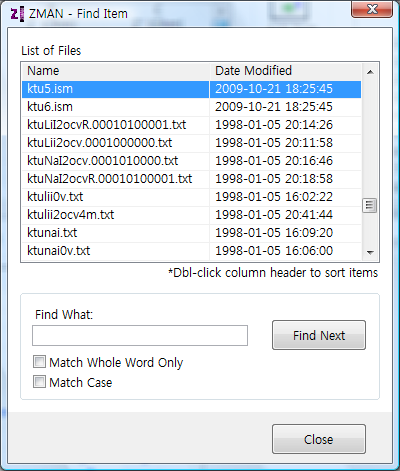Find item menuData replacement by formula functionEmpty cell capacitance calculation (Ver2.2)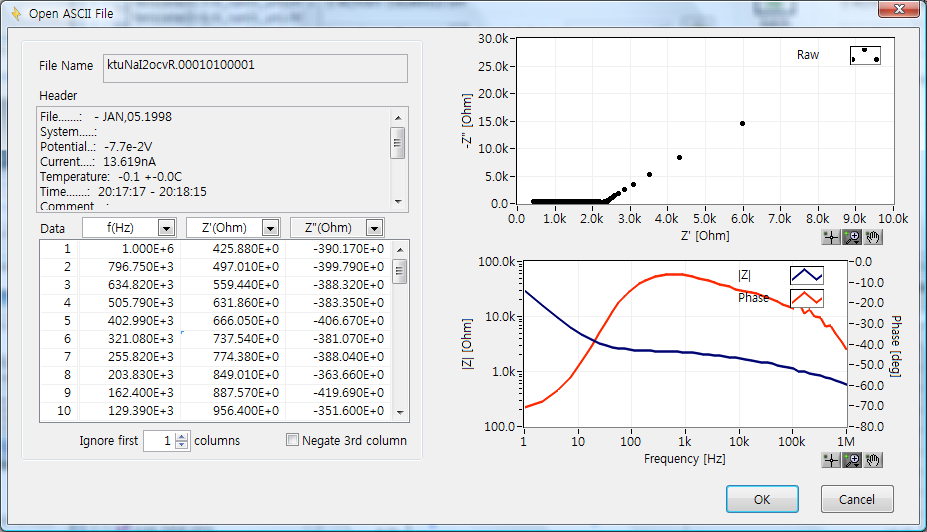Importing 3rd parties ASCII data file (needs license code) (Ver2.2)Project manager/data preview

* Plots

== 2D Nyquist Plot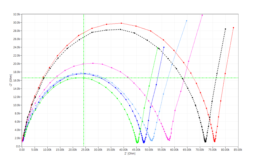2D Nyquist plot setting 2D Nyquist plot

== 2D Bode Plot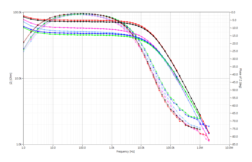2D Bode plot setting 2D Bode plot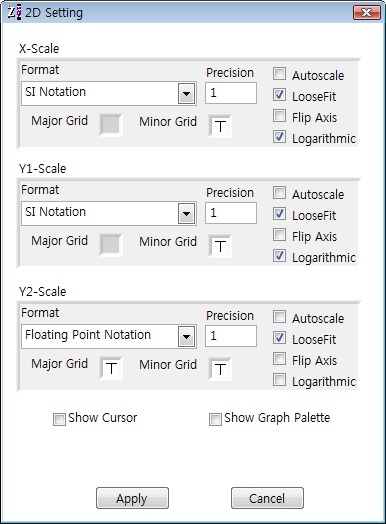2D plot setting

== 3D Plot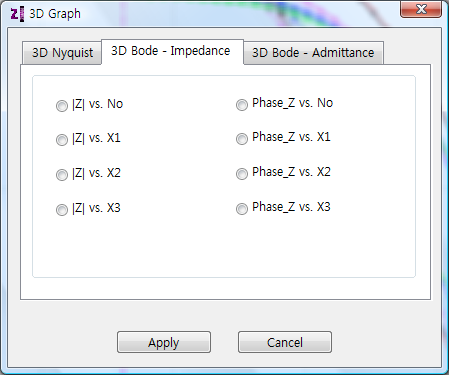3D Nyquist plot setting 3D Bode plot setting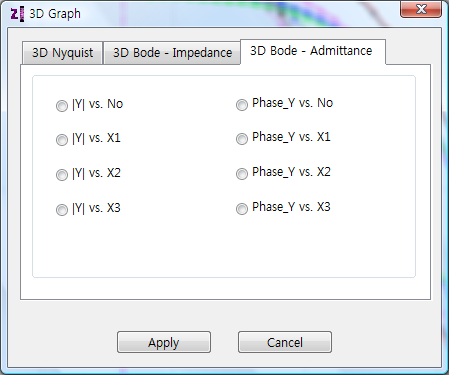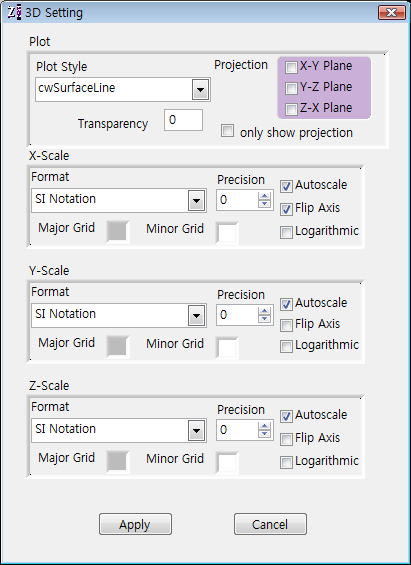3D admittance plot setting 3D plot setting

* Analysis

== Modeling & Fitting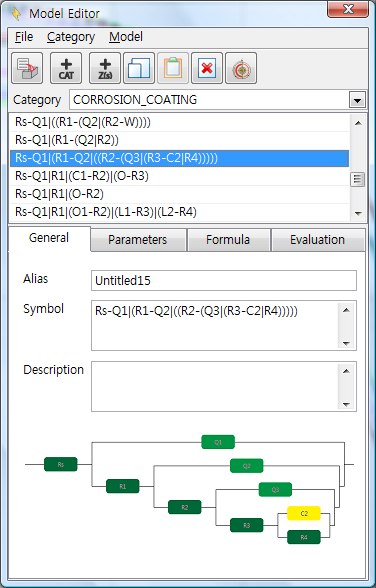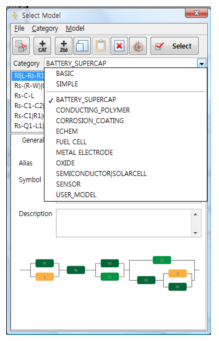Model editor (Ver2.2) Model library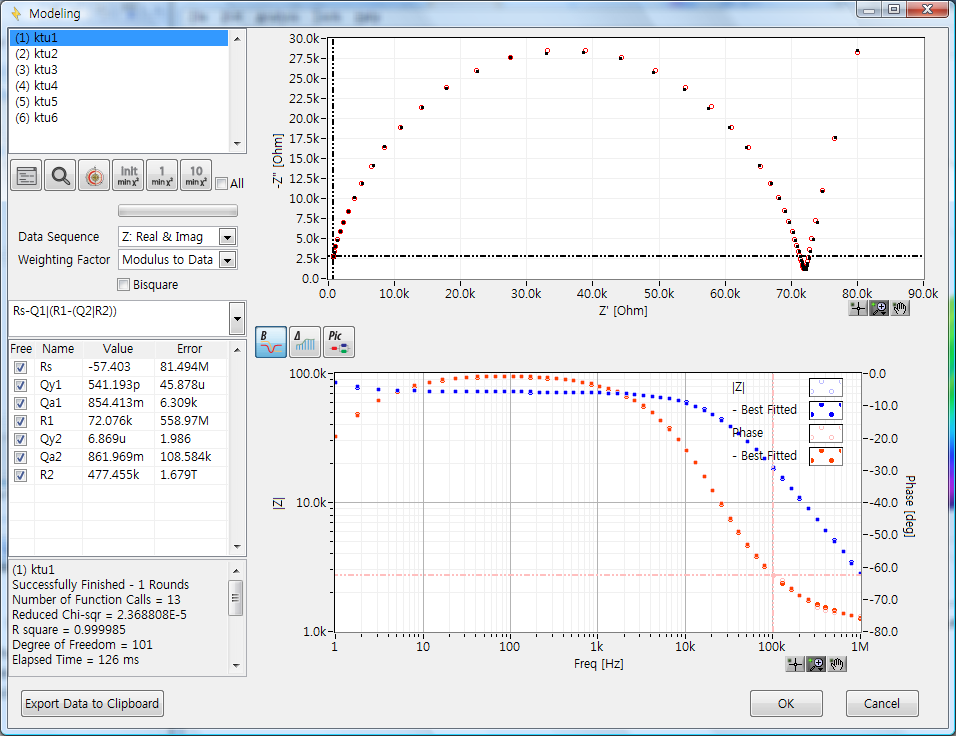LEVM fitting (Ver2.2)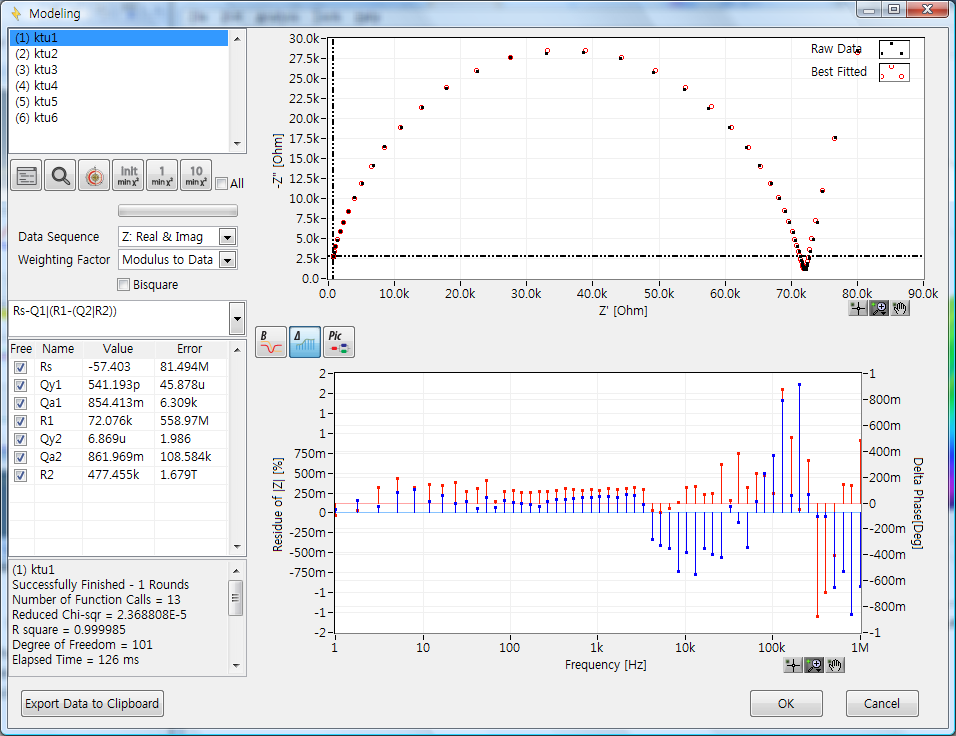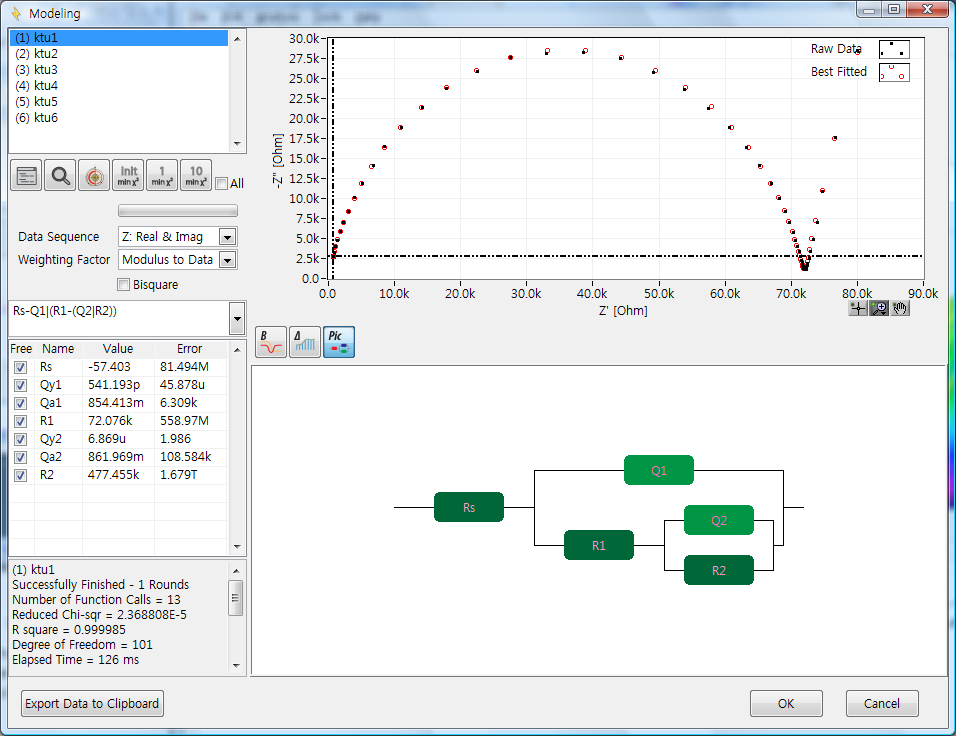Fitting display (Ver2.2)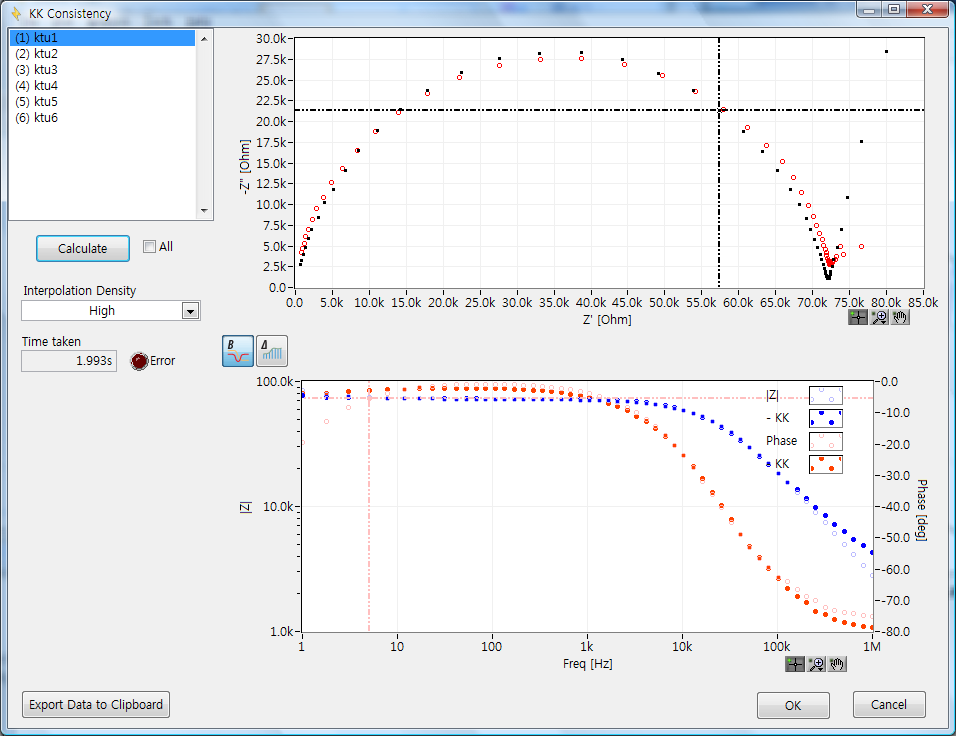KK consistency (Ver2.2)

== 2D Parameter Plot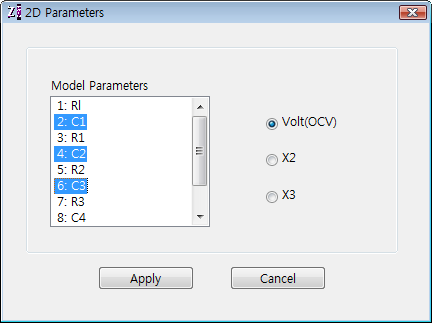Parameter plot setting Parameter plot

== 3D Plot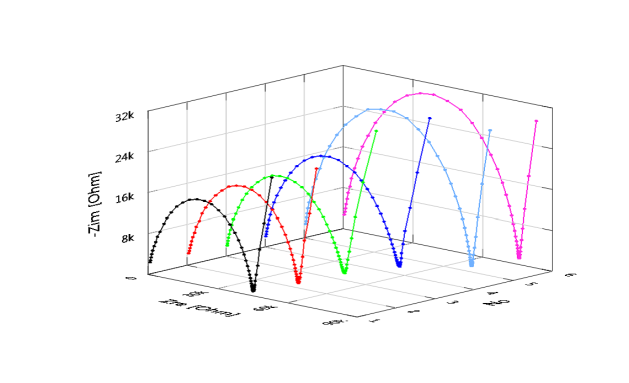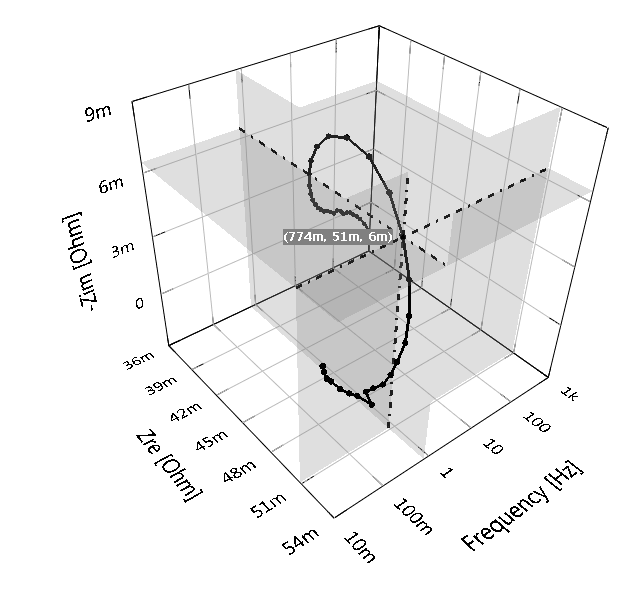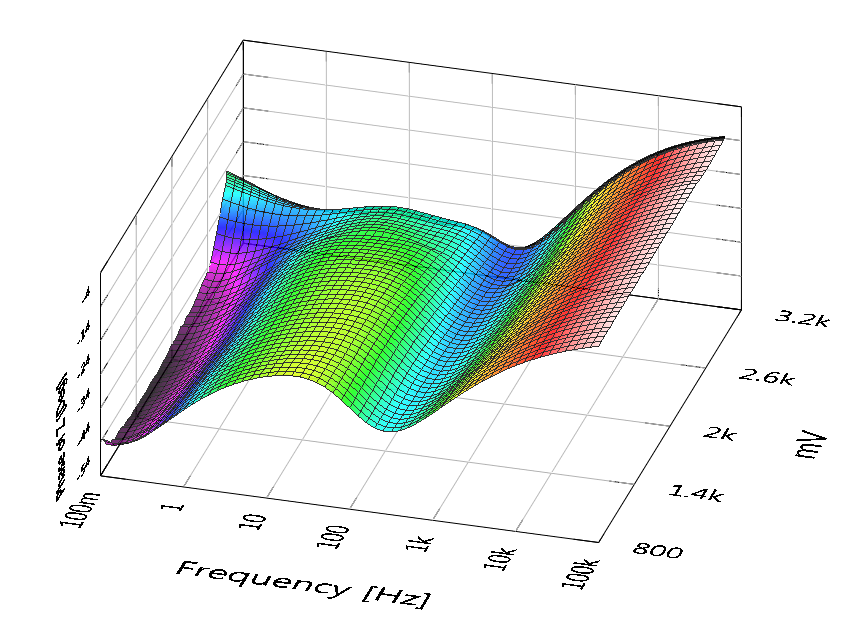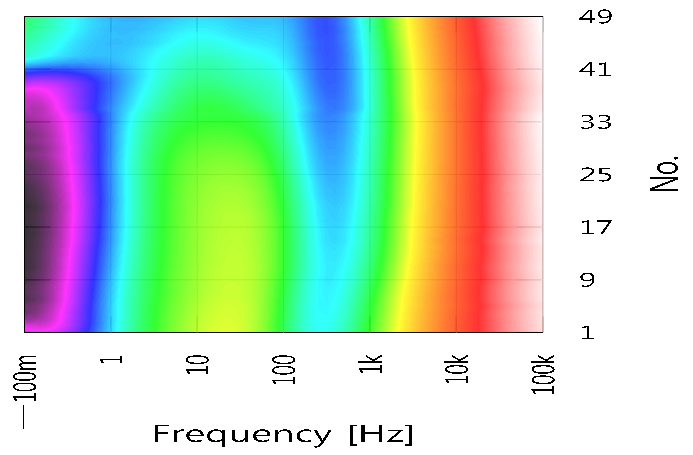© 2011  ZIVE LAB | support@wonatech.com